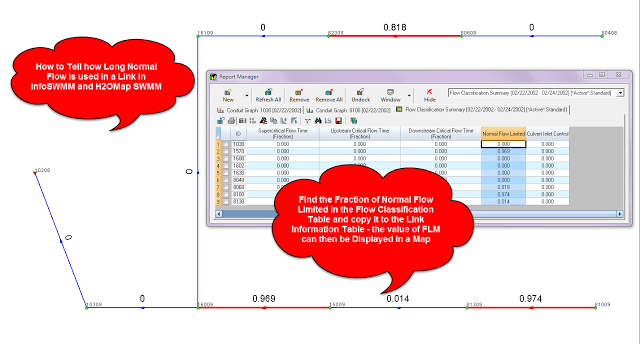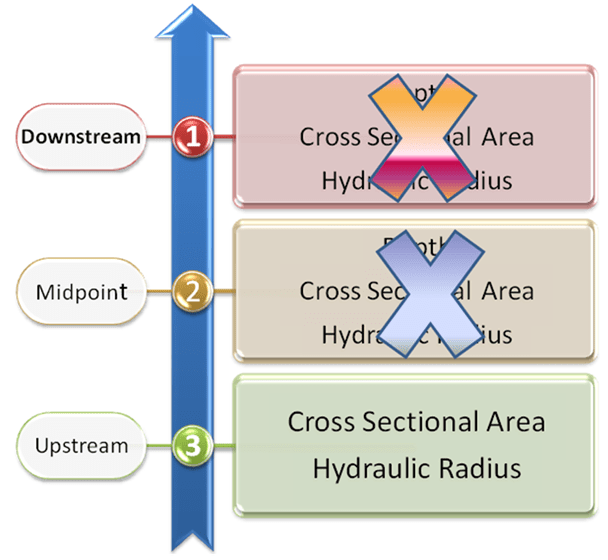# How to Tell how Long Normal Flow is used in a Link in #InfoSWMM, H2OMap SWMM w/ #SWMM5

How to Tell how Long Normal Flow is used in a Link in #InfoSWMM, H2OMap SWMM  w/ #SWMM5

Find the Fraction of Normal Flow Limited in the Flow Classification  Table and copy it to the Link Information Table - the value of FLM can then be Displayed in a Map using  the Map Display command.  In this small example, three links primarily used normal flow and one uses it for a short time. Normal flow only uses the Manning's equation and the link upstream depth, hydraulic radius and cross sectional area to compute the flow.Using Map Display to Show Limited Time St. Venant equation solution – this is the link attribute data used when the St. Venant Equation is used in #SWMM 5. Simulated Parameters from the upstream, midpoint and downstream sections of the link are used.Normal Flow Equation – this is the link attribute data used when the Normal Flow Equation is used in #SWMM 5. Only simulated parameters from the upstream end of the link are used if the normal flow equation is used for the time step.Translate »